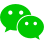• 创建数组
• 数学函数
• 数组索引
• 数组统计
• 数组运算
• 数组切片
• 数组排序

共2个章节

### 实验任务

• 使用 NumPy 创建数组
• 使用 NumPy 对数组进行运算
• 使用 NumPy 对数组进行排序
• 使用 NumPy 对数组形状进行操作
• 使用 NumPy 对数组进行统计

• 本课程难度为简单。

### 面向用户

• 本课程主要讲解 NumPy 的基本操作；该课程适合于对数据分析感兴趣，或者对 NumPy 不熟练的用户。加入社群加入编程交流社群